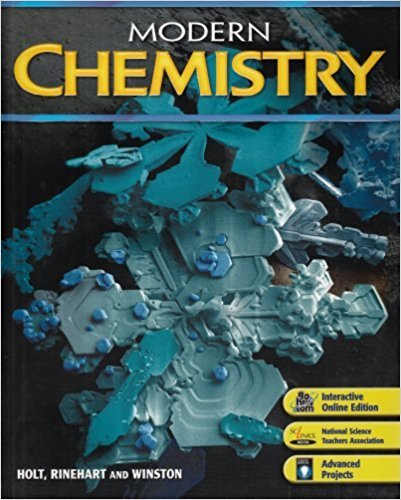Modern Chemistry - 1 Edition - Chapter 11 - Problem 40
Register Now

Join StudySoup

Get Full Access to Modern Chemistry - 1 Edition - Chapter 11 - Problem 409780030735462

# Suppose a 5.00 L sample of 02 at a given temperature and pressure contains 1.08 x 10

Modern Chemistry | 1st Edition

Problem 40

Suppose a 5.00 L sample of 02 at a given temperature and pressure contains 1.08 x 10 molecules. How many molecules would be contained in each of the following at the same temperature and pressure? a. 5.0 L H2 b.5.OLCO2 c. 10.0 L NH3

Accepted Solution
Step-by-Step Solution:
Step 1 of 3

Chemistry 111 lecture notes (Dr. Patton) 4.5- Concentration  Molarity (M in mol/L)  Equation: M=n/V in Liters. You can rearrange this equation to solve for a variety of applications.  V=n/M  n=M x V  EXAMPLE: How many moles of MgCl are present2in 60.0 ml of .100M MgCl 2 solution Convert ml to Liters- 60.0ml (1L/1000ml)= .060 L Find moles using molarity… n=(.100M mol/LMgCl )(.260L)= .006mol  EXAMPLE: How many grams of KCl are present in 250.0 ml of a .125 M solution of KCl Convert ml to Liters- 250.0ml (1L/1000ml)= .250 L Find mass of KCL- 74.55g Find moles of KCl using molarity of solution…

###### Chapter 11, Problem 40 is Solved

Step 2 of 3

Step 3 of 3

Unlock Textbook Solution